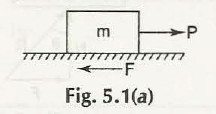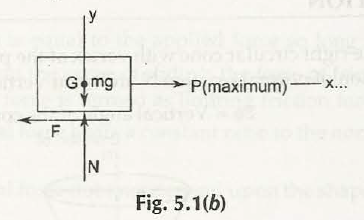Frictional force is something that tries to stop the movements of an object already resting in one condition. This is best understood with one example:

Suppose one solid block of mass or ‘m’ is resting on the rough surface, consider this picture:• The force used to pull that mass is P and in its initial moment that m is unable to move.
• The reason behind this is F which is delivering a counter force to stop m from going into the direction P is pulling.
• The more P tries to pull F increases with each moment.
• You will find this m starts to move or slide on that rough surface only when P pulls with highest force.

Consider this figure:That block of mass will reach equilibrium in this condition.

Let us find the equations to solve this:

First, ∑Fx= 0

P – F =0

F = p

Then, ∑fy= 0

N – mg = 0

N = mg

Links of Next Mechanical Engineering Topics:-### Customer Reviews

My Homework Help
Rated 5.0 out of 5 based on 510 customer reviews at